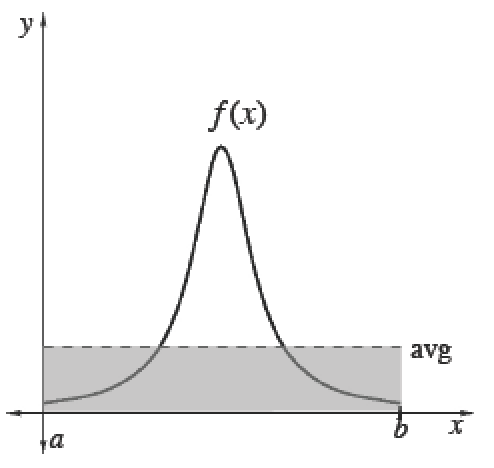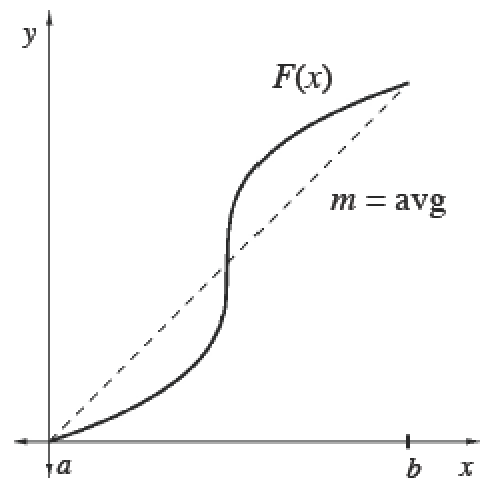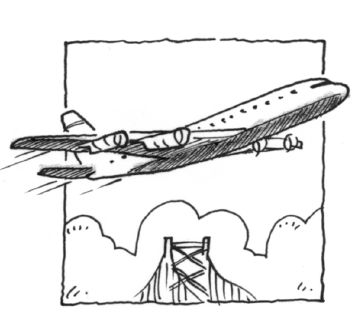### Home > APCALC > Chapter 7 > Lesson 7.2.1 > Problem7-60

7-60.

The velocity of a plane flying from San Francisco is given by $v(t) = 270\sqrt { t }$ where $t$ is measured in hours and $v(t)$ in miles per hour. Calculate the average velocity of the plane between the first and fourth hours.

You are given a velocity function and you want to find average velocity.
Should you use 'average area under the curve' or 'slope of the secant'?

Average (Mean) Values

 To calculate the mean (average) value of a finite set of items, add up the values of items and divide by the number of items.Integrals help us add over a continuous interval. Therefore, for any continuous function $f$ :$\frac{\int_a^bf(x)dx}{b-a}=$ mean value of $f$ over $[a, b]$Since $\int _ { a } ^ { b } f ( x ) d x$, we can also calculate the average value of any function $f$ using its antiderivative $F$. Its average slope gives the average rate of change of $F$, which is the same as the average value of $f.$$\frac{\int_a^bf(x)dx}{b-a}=\frac { F ( b ) - F ( a ) } { b - a }=$  mean rate of change of $F ′$ over $[a, b]$